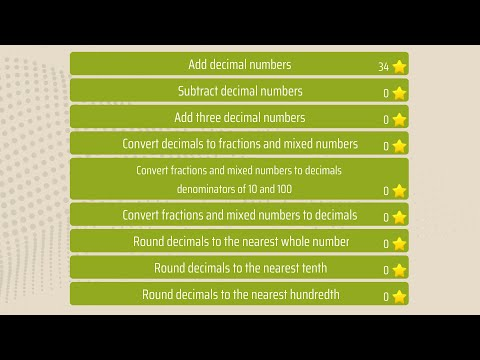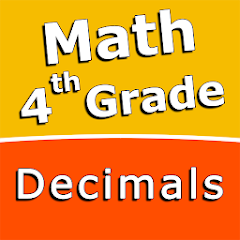# Decimals 4th grade Math skills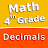1K+Everyone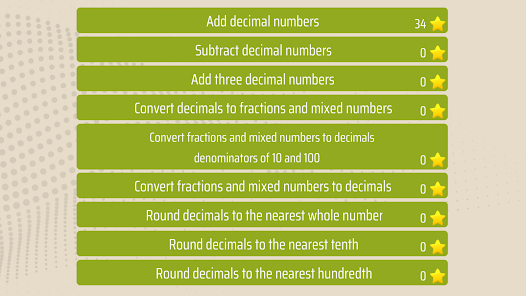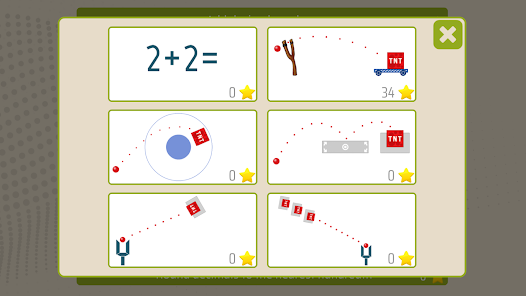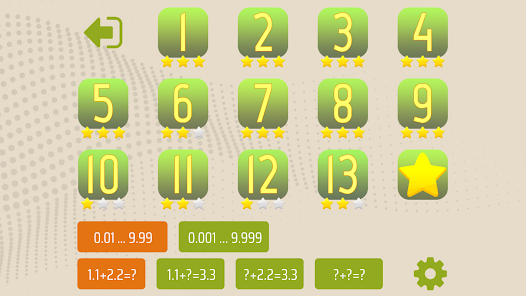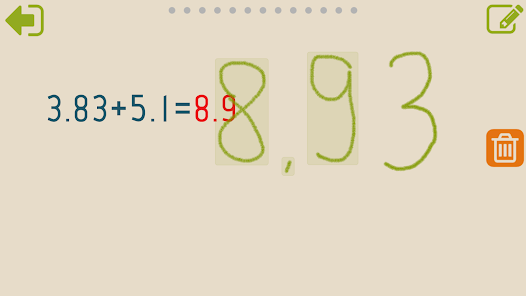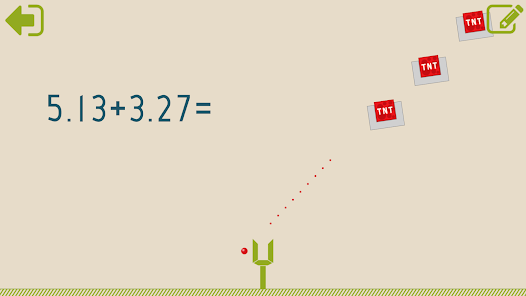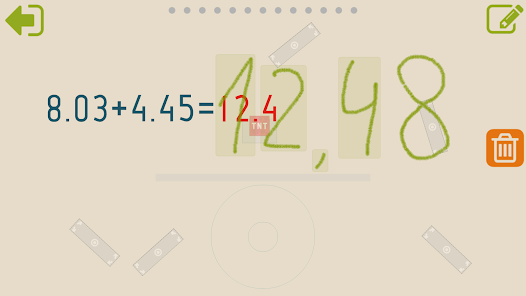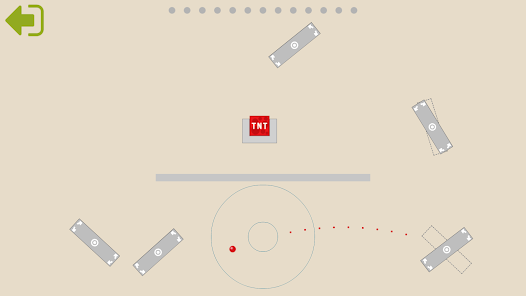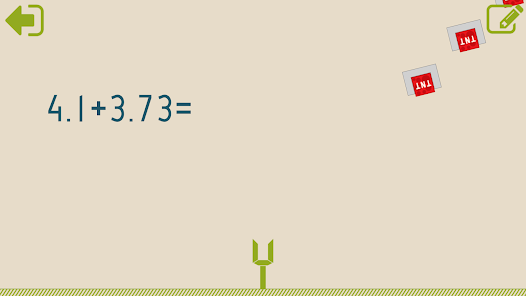Intuitive interface powered by handwriting input and a choice of three fun and engaging mini games in addition to a regular math trainer mode make our app stand out from the crowd of generic math learning apps.

With Fourth grade Math skills - Decimals you can practice and improve the following math skills:
- Subtract decimal numbers
- Convert decimals to fractions and mixed numbers
- Convert fractions and mixed numbers to decimals - denominators of 10 and 100
- Convert fractions and mixed numbers to decimals
- Round decimals to the nearest whole number
- Round decimals to the nearest tenth
- Round decimals to the nearest hundredth
Updated on
Nov 17, 2022

## Data safety

Safety starts with understanding how developers collect and share your data. Data privacy and security practices may vary based on your use, region, and age. The developer provided this information and may update it over time.No data shared with third parties